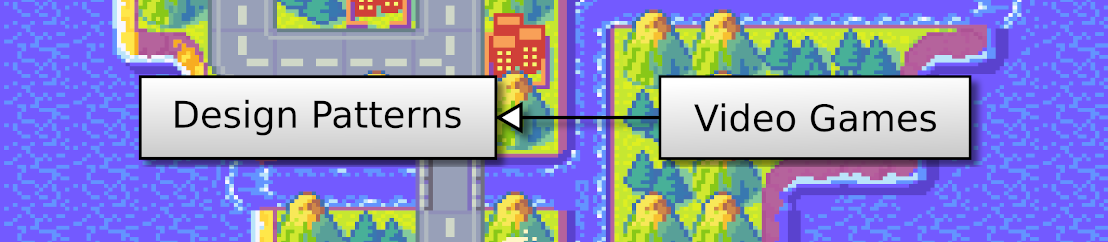## Discover Python and Patterns (15): Lists

I introduce in this post Python lists: with them, we can store and process any number of items, for instance, all the canon towers in our game.

This post is part of the Discover Python and Patterns series

## Lists

Create a list in Python is very easy, for instance, to create an empty list:

``mylist = []``

You can also create a list with an initial content:

``mylist = [12,25,7]``

Lists can contain any objects, and you can mix them:

``mylist = ["Apple",23,True]``

## Access

Items in a Python list are always indexed with a number from 0 to the size of the list minus 1:

``````mylist = [12,25,7]
print(mylist)      # print 12
print(mylist)      # print 25
print(mylist)      # print 7``````

You can see the corresponding value for each index of this list in the following table:

If you try to use an index higher or equal to the size of the list, then Python raises an exception:
``````mylist = [12,25,7]
print(mylist)      # raises an IndexError exception``````

You can also use negative indexes, in which cases you access from the last item to the first one. As for too high indexes, if the index is too small (lower than the opposite of the size), then an exception is also raised:

``````mylist = [12,25,7]
print(mylist[-1])      # print 7
print(mylist[-2])      # print 25
print(mylist[-3])      # print 12
print(mylist[-4])      # raises an IndexError exception``````

## Modification

You can modify any item in the list using the brackets:

``````mylist = [12,25,7]
mylist = 3
print(mylist)       # print [12, 3, 7]``````

As for access, you can't use invalid indexes, e.g. values outside [-size,size-1].

You can add an item using the `append()` method:

``````mylist = [12,25,7]
mylist.append(3)
print(mylist)       # print [12, 25, 7, 3]``````

To remove an item, you can use the `del` statement:

``````mylist = [12,25,7]
del mylist
print(mylist)       # print [12, 7]``````

## Iterate through a list

Like we saw in the previous posts, you can access all items of a list using the `for` statement:

``````mylist = [12,25,7]
for item in mylist:
print(item)``````

These lines print the following messages:

``````12
25
7``````

Note that the iteration is always from index 0 to the last one.

If you need to know the index of each item, you can use the `enumerate()` function:

``````mylist = [12,25,7]
for index,item in enumerate(mylist):
print(index,":",item)``````

These lines print the following messages:

``````0: 12
1: 25
2: 7``````

The iteration through a list is thanks to the Iterator pattern. Once we have seen the class inheritance in another post, I'll show you how to create your iterators.

There are many other features around Python lists, and I'll show them throughout the posts. For now, we have enough knowledge to improve our game.

## Store the towers in a list

In the previous program, we store each tower position in a variable (constructor of the `GameState` class):

``````self.tower1Pos = Vector2(10,3)
self.tower2Pos = Vector2(10,5)``````

We can store the same locations using a list:

``````self.towersPos = [
Vector2(10,3),
Vector2(10,5)
]``````

If you want to add another tower, you only need to add another item to this list, for instance using the `append()`method:

``self.towersPos.append(Vector2(10,4))``

This addition can be in the constructor or any other method of the `GameState` class. For instance, you can add one if you press a key.

All the following no more depends on the number of towers in your game.

## Handle collisions

In the `update()` method of the `GameState` class, we were using a long `if` statement to validate the new tank positions:

``````# Update position only if there is no collisions
if  newTankPos.x >= 0 and newTankPos.x < self.worldSize.x \
and newTankPos.y >= 0 and newTankPos.y < self.worldSize.y \
and newTankPos != self.tower1Pos and newTankPos != self.tower2Pos:
self.tankPos = newTankPos``````

For the locations outside the world, we still use a `if`statement. Note that the following lines use the `return`statement. This statement immediately leaves the current function. It means that if any condition is met (like `newTankPos.x < 0`), then the function execution stops and Python ignores all the following lines:

``````if newTankPos.x < 0 or newTankPos.x >= self.worldSize.x \
or newTankPos.y < 0 or newTankPos.y >= self.worldSize.y:
return``````

If the new tank position is inside the world, the function continues, and we iterate through the tower positions:

``````for position in self.towersPos:
if newTankPos == position:
return``````

For each tower position, if the new tank position is one of a tower, then we also leave the function using the `return` statement.

The final line of the `update()` method is the update of the tank position, which can happen if the position checks all passed:

``self.tankPos = newTankPos``

## Render towers

The rendering of towers is the same as before, except that we have a single block for rendering a tower (cf. `render()` method of the `UserInterface` class). This block is run as many times as there are tower positions in the list:

``````for position in self.gameState.towersPos:
spritePoint = position.elementwise()*self.cellSize
texturePoint = Vector2(0,1).elementwise()*self.cellSize
textureRect = Rect(int(texturePoint.x), int(texturePoint.y), int(self.cellSize.x),int(self.cellSize.y))
self.window.blit(self.unitsTexture,spritePoint,textureRect)
texturePoint = Vector2(0,6).elementwise()*self.cellSize
textureRect = Rect(int(texturePoint.x), int(texturePoint.y), int(self.cellSize.x),int(self.cellSize.y))
self.window.blit(self.unitsTexture,spritePoint,textureRect)``````